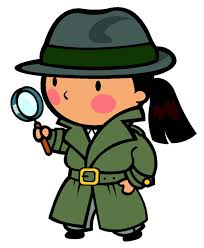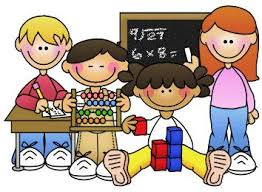# Marie Mizerek's Classroom: We are learning...

Partnering with Parents to Enrich the Student Learning Experience

Phonics:

The Mystery of the Silent eThe goal of the second unit is to build upon the key concepts learned in unit one while continuing to build a community of readers and writers who are interested in how letters, sounds, syllables and word parts work.

Students become word detectives and study words carefully!

Goals include:

• Recognize that some words have long-vowel sounds, represented with a CVCe pattern.
• A long vowel sound may be represented by a vowel team. (CVVC pattern)
• Continue to develop a bank of high-frequency words that can be used to learn more about words.
• Strengthen strategies for breaking words into parts and blending them back together.

## Writing

Writing Unit TwoNonfiction Chapter Books...

Students will plan, sketch, write, edit and revise information books.  Students begin the unit writing basic information picture books and end the unit writing information chapter books.

Students will practice and apply many strategies throughout the unit.  They will self-assess their work guided by a checklist and suggestions from our team members.

We are very excited to write teaching books!

We will learn…

• Write in twin sentences
• Include pictures
• Teaching words
• Lines and arrows
• Zooming in
• Give an example
• Use shape, size and color words
• Use big, bold pop-out words
• Use speech bubbles

## Mathematics

Mathematics Unit OverviewThe unit focuses on understanding addition and subtraction along with applying strategies for solving mathematical problems within 20.

1.OA.1 :Use addition and subtraction within 20 to solve word problems involving situations of adding to, taking from, putting together, taking apart, and comparing, with unknowns in all positions, e.g., by using objects, drawings, and equations with a symbol for the unknown number to represent the problem.

• I can use the numbers 0 to 20 to add and subtract.
• I can solve word problems using manipulatives, drawings and equations.
• I can solve addition and subtraction word problems with numbers 20 and less.
• I can use the numbers 0 to 20 to solve addition, subtraction and comparison word problems with unknown numbers in any position.

1.OA.2: Solve word problems that call for addition of three whole numbers whose sum is less than or equal to 20, e.g., by using objects, drawings, and equations with a symbol for the unknown number to represent the problem.

• I can add three whole numbers whose sum is less than or equal to 20.
• I can solve word problems with three whole numbers using  manipulatives, drawings and equations.
• I can use the numbers 0 to 20 to solve addition word problems with an unknown number.

1.OA.3: Apply properties of operations as strategies to add and subtract.2 Examples: If 8 + 3 = 11 is known, then 3 + 8 = 11 is also known. (Commutative property of addition.) To add 2 + 6 + 4, the second two numbers can be added to make a ten, so 2 + 6 + 4 = 2 + 10 = 12. (Associative property of addition.)

• I can show that adding zero to any number does not change the number.
• I can show that changing the order of the addends does not change the sum.
• I can show that adding three or more numbers in any order does not change the sum.
• I can use the rules of addition and subtraction to add and subtract.

1.OA.4: Understand subtraction as an unknown-addend problem. For example, subtract 10 - 8 by finding the number that makes 10 when added to 8.

• I can rewrite an addition equation as a subtraction equation.
• I can rewrite a subtraction equation as an addition equation.
• I can use fact families to show the relationship between addition and subtraction.
• I can rewrite a subtraction equation as an addition equation with a missing addend.

1.OA.5: Relate counting to addition and subtraction (e.g., by counting on 2 to add 2).

• I can count up to add.
• I can count back to subtract.
• I can count by 2's, 5's and 10's.
• I can use skip counting to add and subtract.

1.OA.6: Add and subtract within 20, demonstrating fluency for addition and subtraction within 10. Use strategies such as counting on; making ten (e.g., 8 + 6 = 8 + 2 + 4 = 10 + 4 = 14); decomposing a number leading to a ten (e.g., 13 - 4 = 13 - 3 - 1 = 10 - 1 = 9); using the relationship between addition and subtraction (e.g., knowing that 8 + 4 = 12, one knows 12 - 8 = 4); and creating equivalent but easier or known sums (e.g., adding 6 + 7 by creating the known equivalent 6 + 6 + 1 = 12 + 1 = 13).

• I can solve addition and subtraction equations within 10 automatically.
• I can use fact families to solve addition and subtraction equations.
• I can use known facts (plus one (+1), plus two (+2), double facts and sums of 10) to solve more difficult addition and subtraction equations.

1.OA.7:  Understand the meaning of the equal sign, and determine if equations involving addition and subtraction are true or false. For example, which of the following equations are true and which are false? 6 = 6, 7 = 8 - 1, 5 + 2 = 2 + 5, 4 + 1 = 5 + 2.

• I can explain that the equal sign means “the  same as”.
• I can compare the value of both sides of an equation and tell if the equation is true or false.

1.OA.8: Determine the unknown whole number in an addition or subtraction equation relating three whole numbers. For example, determine the unknown number that makes the equation true in each of the equations 8 + ? = 11, 5 = _ - 3, 6 + 6 = _.

• I can solve addition equations that have an unknown number.
• I can solve subtraction equations that have an unknown number.